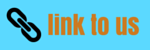# Rotation

## (9) Torque and angular acceleration

• While discussing and defining torque or moment of force ,we found that necessary condition for a body not to rotate is that resultant torque about any point should be zero
• However this condition is necessary but not sufficient for a rigid body to be static for example in absence of resultant torque a body once set in rotation will continue to rotate with constant angular velocity
• Analogous to translation motion when torque acts on a rigid body rotating about a point with constant angular velocity then angular velocity of the body does not remain constant but changes with angular acceleration α which is proportional to the externally applied torque
• Consider a force Fi acting on the ith particle of mass mi of the rigid body pivoted about an axis through point O as shown below in the figure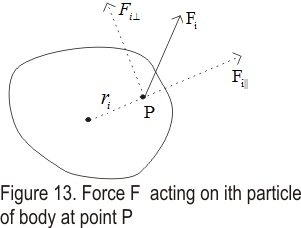• This force Fi as discussed earlier has two components one parallel to the radius vector ri and one perpendicular to the ri
• Component of force parallel to radius vector does not have any effect on the rotation of the body
• Component of force Fi perpendicular does affect the rotation of the body and produces torque about point O through which the body is pivoted which is given by
τi=Fi⊥ri ---(21)
• if Fi⊥ is the resultant force acting on the ith particle ,then from Newton�s second law of motion
Fi⊥=miai⊥ = miriα ----(22)
where ai⊥ is the tangential acceleration of the body
• From equation (21) and (22)
τi=miri2α
And taking sum over all the particles in the body we have
∑τi=∑(miri2α)=α∑(miri2) ---(23)
as angular acceleration is same for all the particles of the body
• we know that
∑(miri2) =I
where I is the moment of inertia of the rigid body .Hence in terms of moment of inertia equation 23 becomes
∑τ=Iα                      ---(24)
we have denoted resultant torque acting on the body ∑τsub>i as ∑τ
• Both the torque and angular acceleration are vector quantities so in vector form
τ=Iα                ---(25)
• Alternatively equation (24) which is rotational analogue of Newton second law of motion ( ∑F=ma) can be written as
τ=Iα = I(dω/dt)=d(Iω)/dt                      ---(26)
which is similar to the equation
F=d(mv/dt=dp/dt
where p is the linear momentum
• The quantity Iω is defined as the angular momentum of the system of particles
Angular momentum =Iω
L=Iω
• From equation 26 we see that resultant torque acting on a system of particles equal to the rate of change of the angular momentum
τ=dL/dt

## (10) Angular momentum and torque as vector product

(A) Angular momentum
• In any inertial frame of refrance the moment of linear momentum of a particle is known as angular momentum or, angular momentum of a particle is defined as the moment of its linear momentul.
• In rotational motion angular momentum has the same significance as linear momentum have in the linear motion of a particle.
• Value of angular momentum of angular momentum is equal to the product of linear momentum and p(=mv) and the position vector r of the particle from origin of axis of rotation.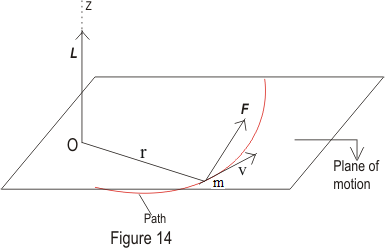• Angular mmomentum vector is usually represented by L.
• If the linear momentum of any particle is p=mv and its position vector from any constant point be r then abgular momentum of the particle is given by
L = r×p = m(r×v)               (1)
• Angular momentum is a vector quantity and its direction is perpandicular to the direction of r and p and could be found out by right hand screw rule.
• From equation 1 scalar value or magnitude of angular momentum is given as
|L|=rpsinθ                    (2)
where V is the angle between r and p.
• For a particle moving in a circular path
v=ω×r;                    (3)
where ω is the angular velocity.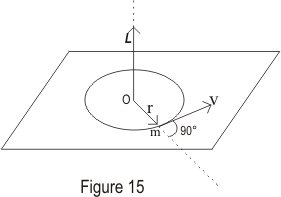Therefore
L=m[r×(ω×r)] = m{ω(r.r)-r(r.ω)} = mr2ω=Iω;                    (4)
(r.ω)=0 because in circular motion r and ω are perpandicular to each other. Here I is the moment of inertia of the particle about the given axis also the direction of L and ω is same and this is a axial vector.
writing equation 1 in the component form we get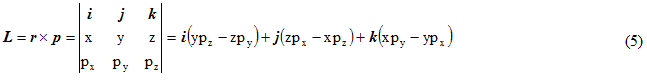• Writing angular momentum in component form we get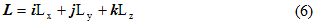writing equation 5 again we get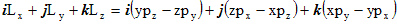Comparing unit vectors on both the sides we get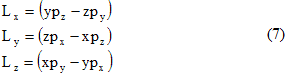• Unit of angular momentum in CGS is gm.cm2/sec and in MKS system it is Kgm.m2/sec or Joule/sec.
(B) Torque
• The turning effect of the force about the axis of rotation is called the moment of force or torque..
• In rotational motion torque has same importance as that of force in the linear motion.
• Torque due to a force F is measured as a vector product of force F and position vector r of line of action of force from the axis of rotation.
• We already know that orque is denoted by letter τ.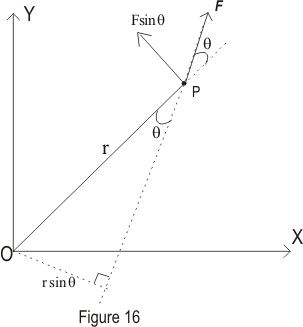• If F is the force acting on the particle and r is the position vector of particle with respect to constant point then the torque acting on the particle is given by
τ=r×F                         (8)
• FRom equation 8 magnitude or resultant of torque is given by
|τ|=rfsinθ                         (9)
where θ is the angle between r and F.
• From equation 9 if θ=900 this menas r is perpandicular to F then,
• FRom equation 8 magnitude or resultant of torque is given by
|τ|=rF
and if θ=00 this menas r is parallel to F then,
|τ|=0
• Unit of torque is Dyne-cm or Newton-m
(C) Relation between angular momentum and torque
• Differentiating equation 1 w.r.t. t we get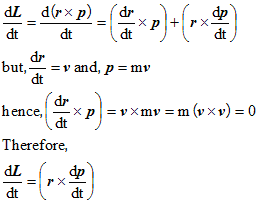• But from Newton's second law of motion we have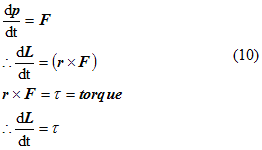Hence rate of change of angular momentum with time is equal to the torque of the force.